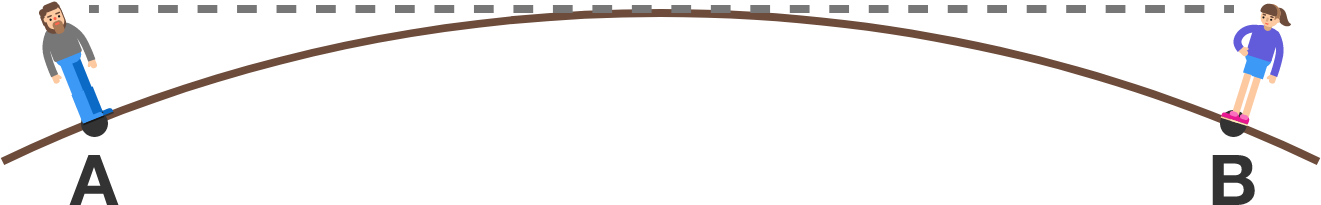# Trying to prove the earth is flat

Geometry Level 1

In 1838, Samuel Birley Rowbotham conducted observations leading him to conclude the earth was flat. His idea was the following: If light goes straight and I'm standing at $A$ with my friend, there must be a point $B$ where I can't see him.

Assuming light does not bend because of atmospheric refraction, which of these is closest to the minimum arc length of $\stackrel\frown{AB}$ that would prevent Samuel from seeing his friend? Assume they are both $1.8$ meters tall and the radius of the earth is $6,371,009\text{ m}.$Note: Though the earth is (of course) not flat, Samuel was able to see his friend at a farther distance than he was supposed to. This is due to atmospheric refraction, i.e. the non-homogeneous density of air creating a slight deviation of light. Without knowing this effect, a newspaper editor performed in 1896 the same experiment and concluded the earth was concavely curved.

×